## Tuesday, September 23, 2008

### Number Theory with Mathematica

You can find the original post on the Dead Engineers Society.

Cite as:

## Saturday, September 20, 2008

### CNN Videos and Firefox

For some reason, CNN videos do not play in Firefox 3.0. Ive done some search over this, but couldn't find the reason for this behaviour. However, it seems that clearing your Firefox cache makes things work!

Here's how
In Firefox, got to Tools/Clear Private Data
alternatively, if you want to control what is being cleared (i.e. clearing only the cache not the saved passwords etc..)
Tools/Options/Privacy and make sure you select "Aske me before clearing private data"
With this option enabled, every time you clear private data, you will get a popup window asking you what you want to clear.

Voila!

Cite as:

## Tuesday, September 16, 2008

### 5. The Reynolds Transport Theorem

[Previous Article: The Material Derivative in Vector Form]

For completion purposes, I feel obliged to discuss the Reynolds transport theorem. Although I would like to derive the fluid flow equations from scratch, the Reynolds transport theorem provides an avenue for a simple way to derive them. Henceforth, I decided to use the many ways of deriving the conservation equations, whether in integral or differential form.

I will base the current derivation on the text by James A. Fay Introduction to Fluid Mechanics. I believe it is an excellent text on fluid mechanics that focuses on the essential physics of fluid flows.

We first have to distinguish between a material volume and a control volume. A material volume is a volume of fluid that contains the same fluid as it moves and deforms in time.

A control volume is a fixed volume in space where the fluid passes through.

This is tightly linked to the previous discussions on the material derivative and its connection with the Lagrangian and Eulerian views. A material volume is part of a Lagrangian description whereas a control volume is part of the Eulerian description.

Now let us consider and "extensive" property B whose "intensive" property is b. For example, mass is an extensive property, whereas the density is the corresponding intensive property. An extensive property describes a specific part of the fluid (e.g. the mass is different for different volumes of the same fluid) while the intensive property is intrinsic (e.g. the density is the same for different volumes of the same fluid). In simple terms, an intensive property is the extensive property per unit mass.

The Reynolds transport theorem can be thought of as the integral form of the material derivative. It mainly relates the rate of change of an extensive property of a given material volume to the rate of change of the corresponding intensive property.

The total amount of property B in a given material volume is therefore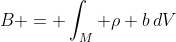(Eq. 1)

As the material volume moves around, the quantity B inside M changes due to external forces or internal reactions for example. Therefore, it is convenient to compute the time rate of change of B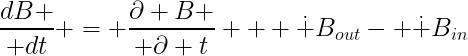(Eq. 2)

Eq. 2 means that the rate of change of the quantity B in the material volume is equal to the rate of change of B within the fixed control volume plus the net flowrate of the quanity B through the control surface. The RHS of Eq. 2 can be expressed as follows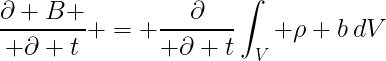(Eq. 3)
and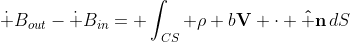(Eq. 4)

Eq. 4 measures the flux of the quantity B through the control surface. Then, combining the above equations, we get the Reynolds transport theorem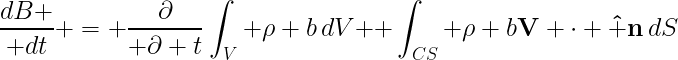(Eq. 5)

Voila!

There is an alternative way of deriving the Reynolds transport theorem, however, it makes use of the continuity equation which we have not derived yet. So this will be postponed to a later post.

[Next Article: How Euler Derived the Continuity Equation]

Cite as:

### 4. The Material Derivative in Vector Form

[Previous Article: 3. The Material Derivative in Spherical Coordinates]

After going over the derivation of the material derivative in Cartesian, cylindrical, and spherical coordinates and seeing all the trouble that we had to go through, it is time to present the material derivative in a vector invariant form.

An invariant vector form is independent of the coordinate system used. The form will be the same for all coordinate. Of course, vector operations (i.e. gradient and curl) are not the same for different coordinate systems [see this post] once they are expanded; but the gradient is always a gradient and the curl is always a curl - only the expansion is different.

I will follow Karamcheti's explanation for obtaining the vector form of the material derivative. We start by considering a fluid particle at R measured from the origin of the coordinate system and time t. Consider also a generic scalar fluid property Q such as the temperature, pressure, or density. The scalar restriction will be removed once we obtain the general form for the material derivative. At point R and time t, the property is defined as Q(R, t). At time (t + Δt), the fluid particle moves a distance Ds and the fluid property changes accordingly to Q(R + V Δt, t + Δt)

The total change in Q from t to (t + Δt) is

If we substitute Eq. 3 into Eq. 2, we get the following(Eq. 4)

Note that all high order terms disappear as the limit in the derivative is applied. The second term in Eq. 4 can be cast in vector form because it represents the derivative of Q in the direction of the streamline, tangent to the velocity vector. This means that it can be written as the dot product of the gradient of Q and the unit vector along the streamline, i.e. parallel to the velocity. Mathematically, this can be written as(Eq. 5)

at the outset, we recover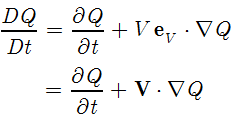(Eq. 6)

Voila!

This is the expression we are looking for; Eq. 6 represents the time derivative of a transported fluid property as seen from an Eulerian point of view. This also works when Q is a vector field, call it A(Eq. 7)

However, the form given by Eq. 7 only works for Cartesian coordinates because it not invariant under coordinate transformation. This means that it does not hold true when using curvilinear coordinates such as Cylindrical or Spherical. Fortunately, we can write it using invariant form as follows

Cite as:
Saad, T. "4. The Material Derivative in Vector Form". Weblog entry from Please Make A Note. https://pleasemakeanote.blogspot.com/2008/08/derivation-of-navier-stokes-equations_20.html

### 3. The Material Derivative in Spherical Coordinates

Spherical coordinates are of course the most intimidating for the untrained eye. For engineers and fluid dynamicists, the farthest we go is usually cylindrical coordinates with rare pop-ups of the spherical problem. Here, I want to derive the material derivative of the velocity field in spherical coordinates. First, let us do that for a scalar.

Assume that at point r and time t a fluid particle has a property Q. As this particle moves about, this property will change with time (and space). Again, in the Lagrangian description, Q is only a function of time, i.e.(Eq. 1)

However, from the Eulerian point of view, any property of the fluid is a function of time and space, which is also a function of time implicitly. Then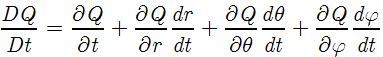(Eq. 3)

where we have used the chain rule to account for the spatial dependence on time. Remembering some of the formulas from dynamics, we have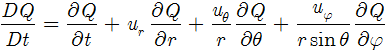(Eq. 5)

To obtain the material derivative for a vector field, we follow a similar procedure keeping in mind the directional nature of a vector. We illustrate this using the velocity field - keep in mind that this works for any kind of vector field. Again, in a Lagrangian reference, the velocity is only a function of time. In the Eulerian view, the velocity has the following form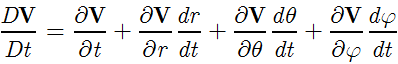(Eq. 7)

Again, noting that the partial derivative with respect to time in Eq. 7 (first term) is evaluated at a fixed position in space, the unit vectors associated with the fluid particle at that point are fixed as viewed from an Eulerian reference, therefore,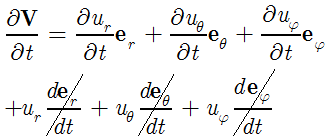(Eq. 8)

To evaluate the remaining terms in Eq. 7, we have to first remember some equations from dynamics or vector calculus about differential changes in unit vectors in spherical coordinates. These are given by(Eq. 12)

Voila!!!

Once Eqs. 8 through 12 are put together, one obtains the full expression for the material derivative of a vector field in spherical coordinates.

In the next post, I will present an invariant vector form for the material derivative so that we don't have to go through all the hassle of using chain rule differentiation to evaluate the material derivative. But it was worth it to see how it works using good old calculus.

Cite as:
Saad, T. "3. The Material Derivative in Spherical Coordinates". Weblog entry from Please Make A Note. https://pleasemakeanote.blogspot.com/2008/08/derivation-of-navier-stokes-equations_18.html

### 2. The Material Derivative in Cylindrical Coordinates

This one a little bit more involved than the Cartesian derivation. The reason for this is that the unit vectors in cylindrical coordinates change direction when the particle is moving.

In the Lagrangian reference, the velocity is only a function of time. When we switch to the Eulerian reference, the velocity becomes a function of position, which, implicitly, is a function of time as well as viewed from the Eulerian reference. Then

and the material derivative is written as (with the capital D symbol to distinguish it from the total and partial derivatives)

Special attention must be made in evaluating the time derivative in Eq. 2. In dynamics, when differentiating the velocity vector in cylindrical coordinates, the unit vectors must also be differentiated with respect to time. In this case, the partial derivative is computed at a fixed position and therefore, the unit vectors are "fixed" in time and their time derivatives are identically zero. Then, we have

we can now evaluate the remaining terms in Eq. 2 as follows

finally(Eq. 6)

When these are put together, the material derivative in cylindrical coordinates becomes(Eq. 7)

This was a rather tedious way of deriving the material derivative as one could have used vector technology to obtain an invariant form that works for all coordinates. Nonetheless, it is interesting to see the intricacies of the derivation using chain rule differentiation. Note that if were computing the material derivative for a scalar, the extra terms in Eq. 7 (in the radial and tangential components) would disappear. These are purely reminicsent of the vectorial nature of the velocity field (or any other vector field for that matter).

It is very interesting to note the intimate link between the physical nature of the velocity and its mathematical description through vectors. One would pose the following argument: why don't we treat the material derivative of the velocity as that of three scalars, namely, u_r, u_theta, and u_z? Doing this will obviously remove the hassles of dealing with derivatives of unit vectors, but will eventually lead to inconsistent results. So what's the issue here?

The problem with that treatment is that in essense, the velocity is one quantity that we describe using vectors: a magnitude and a direction. If we are to use three scalars to describe the velocity we lose an essential ingredient which is the direction. In the end, the material derivative of the velocity can be decomposed into the material derivatives of three scalars (u_r, u_theta, and u_z) plus some correction. This correction stems from the directional nature of the velocity field. In other words, this correction can be thoguht of as being the material derivative of the direction of the velocity field.

[Next Article: The Material Derivative in Spherical Coordinates]

Cite as:
Saad, T. "2. The Material Derivative in Cylindrical Coordinates". Weblog entry from Please Make A Note. https://pleasemakeanote.blogspot.com/2008/08/derivation-of-navier-stokes-equations_17.html

### 1. The Material Derivative in Cartesian Coordinates

Recently, we've had some controversy about some terms in a momentum equation in one of the papers that one of my colleagues is studying. Indeed, for compressible and non constant viscosity fluids, it is not straightforward to come up with the equations of motion as this situation is rarely the case in our daily engineering investigations.

As the problems we are dealing become more complicated (in fact, we add the complication as we solve the problem one step at a time. For example, we start with an inviscid incompressible model, then we add viscosity, then we add compressibility to the inviscid model, then add viscosity to the compressible model etc...).

So anyway, I decided to once and for all settle down the issue and put together a convenient and easy to understand post.

The Material Derivative

First, let us start by going over the material derivative one more time. As you most probably know, there are two reference frames that can be used in studying fluid motion; namely, the Lagrangian and Eulerian frames.

In the Lagrangian description, each particle in the fluid is followed as if it were a "rigid body". This means that each particle has a unique identifier or tag. This description is akin to rigid body dynamics and is a great way of describing a relatively small number of particles. However, when dealing with a fluid, there is a very large number of particles thus rendering a Lagrangian description of a fluid flow problem very tedious and intractable.

In the Eulerian description, flow properties (velocity, pressure, temperature, etc...) are defined as a function of space and time. This means that instead of tagging each particle of the flow, the viewer fixes a volume in space and identifies the flow properties in that region of space. Therefore, it does not matter which particle passes through the volume since that particle will assume the flow property of that point in space.

The natural way of defining the velocity or acceleration is based on a Lagrangian description because it is the easiest (and that's how we've done it in dynamics in high school)! When it comes to fluid flow, the acceleration of a fluid particle as seen in the Eulerian reference is different from the Lagrangian description. This is due to the fact that as the particle moves about, its velocity and position change as well. In Mathematical terms, the particle velocity is

of course, the velocity is a vector

Now the accelerationo is obtained by differentiating the velocity with respect to time. But since the velocity is a function of the position, and the position is a function of time, then we have to use implicit or chain rule differentiation as follows(Eq. 3)

which yields the following expression for the acceleration of ANY fluid particle as seen by an observer in an Eulerian reference.

If we were in a Lagrangian frame, then the velocity is only a function of time because in that reference, the observer is riding on the particle as it flows through space.

[Next Article: The Material Derivative in Cylindrical Coordinates]

Cite as:
Saad, T. "1. The Material Derivative in Cartesian Coordinates". Weblog entry from Please Make A Note. https://pleasemakeanote.blogspot.com/2008/08/derivation-of-navier-stokes-equations.html

## Monday, September 15, 2008

### How to Disable the Firefox Site Specific Zoom

Firefox 3.0 has the ability to keep track of the zoom level you have chosen for a given website. To cancel this feature do the following
1. Type about:config in the Firefox toolbar
2. Locate browser.zoom.siteSpecific
3. Double click on that entry to change value from "true" to "false"
Voila!

Thanks to My Digital Life for sharing this info.

Cite as:
Saad, T. "How to Disable the Firefox Site Specific Zoom". Weblog entry from Please Make A Note. https://pleasemakeanote.blogspot.com/2008/09/how-to-disable-firefox-site-specific.html

### Firefox Blurry Images

Reset the zoom level for that domain
Ctrl + 0 (zero)
I recently faced a weird issue with Firefox. All the images on my blog were rather blurry as if they were wrongly scaled. So I tried with a new Firefox profile and it worked fine. So there must have been some setting or some add-on that is part of the current user profile that is causing this. I removed all the extensions and add-ons to no avail. Just moments ago, I found the solution on the Blitz Research forum. It turns out to be an issue related to the zoom level for a given website, which Firefox 3.0 remembers. By clicking Ctrl + 0, you reset to the no zoom.

Cite as:

## Tuesday, September 9, 2008

### How to Post Math Equations in Blogger Using Latex

In a previous post, I discussed how to post math equations in blogger using the ASCIIMathML script. The advantage of using this method is that you are not dependent on any external server to host your equations since they are NOT images. ASCIIMathML displays equations in ASCII format, i.e. in plain text format.

The bottleneck of this method, as I discovered later on, is its limited capability to display some complicated mathematical forms. The famous example is the limit as x goes to zero. This is how it looks using ASCIIMathML

$\lim_{x\rightarrow 0}$

I was glad to find these limitations before engaging in posting topics related mathematics. It would have been a mess to go back and forth and convert equations etc...

The method that I will discuss today is an old technique that I passed upon when I first started this blog, but had completely neglected. It uses LaTeX to display equations. Of course, this is a good sign. But how does it work? Very simple.

There is a server somewhere over the internet that has "software like" capabilities, i.e. it can process user requests dynamically. These capabilities include Perl,PhP, ASP etc...

On this server, there would be an application that takes a LaTeX syntax as input, processes it on the server, and converts it to an image on the fly.

Voila!

But where is that server? Who wrote that application? Where are the images hosted? Will the application be there, forever?

One question at a time.

Where is that server?
There are several servers out there, the ones I know of are http://www.codecogs.com/ and http://www.forkosh.com/ (which uses mimeTEX).

Who wrote that application?
One, two, or more programmers.

The CodeCogs application was written by Will Bateman and Steve Mayer and can be accessed at:
http://www.codecogs.com/gif.latex?

The mimeTEX interpreter was written by John Forkosh and can be accessed at:
http://www.forkosh.dreamhost.com/mimetex.cgi?

Where are the images hosted?
On the server, temporarily. Due to the dynamic nature of the LaTeX interpreters, they are converted to an image whenever a webpage posts a request. For example, whenever you load a page that has an equation, the server immediately interprets that equation, converts it to an image and sends back that image. I assume that there would be a cached version of the image on the server that is deleted after some time.

Will the application be there forever?
I hope so! The only alternative is to host that application yourself, on your own server. But hey! Can you make sure that you will be able to pay for that hosting for the rest of your life? This dependence on an external server is no different than our depends on google. As for the equations, I am currently hosting them on Picasa, which is basically like hosting the equation on an external server. But, remember, all you need is the application that interprets LaTeX, not the images! So, in the event that one of these applications disappears, you can very simply point to another application on some other server. It is very simple as you will see shortly.

So how does it work in blogger?

You simply create an img tag and set the source of the image as the location of the application followed by the LaTeX syntax. Here's an example. Let's say you want to write this equation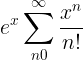The LaTeX syntax is

e^x = \sum\limits_{n = 0}^\infty {\frac{{x^n }}{{n!}}}

create an img tag with the appropriate src field pointing to the online LaTeX renderer and then insert the LaTeX code, i.e.

<img src="http://www.codecogs.com/gif.latex?e^x = \sum\limits_{n = 0}^\infty {\frac{{x^n }}{{n!}}}" />
Voila!

Of course, this is a mess! If everytime you want to write an equation you have to write down the img tags and insert the path to the LaTeX renderer... that is very inefficient!

Fortunately, there are several ways to overcome this. The first one, thanks to the guys at codecogs, their online LaTeX editor prepares the img block for you. All you have to do is
1. Go to the codecogs online LaTeX editor
2. Type in your equations LaTeX
3. Copy the HTML code that is generated at the bottom of the page
4. Paste it in Blogger (either in the "Edit HTML" or "Compose views).
Voila!

After the equation is displayed, you can align it the way you want using the options provided by the blogger editor. The images generated by the codecogs LaTeX editor are transparent with a black foreground. In case your blog has a dark background, they won't look nice at all. What you have to do in this case (which is what I am doing on this blog) is create a new class for images that has a white background. Here's what you need to do
1. Go to your blog's Layout
2. Click on "Edit HTML"
3. Place your class somewhere before ]]> </b:skin >
4. Define your new class as follows:
img.latexEquation {
background:white;
margin: 0px;
}
5. When you paste the HTML code from codecogs, specify which class is applied to the image as follows:
<img class="latexEquation" src="..."/>
Voila!

Now your equations will have a nice white background behind them.

Alternatively, you can use the mimetex editor which gives you the option to make an opaque background and reverse the colors for blogs with a dark background. Make sure you specify \opaque for an opaque background and \reverse for a white foreground. \reverse\opaque will set a black background and white foreground. Here's how it looks with the \reverse\opaque settingand with the \reverse only[To be continued...]

Cite as:
Saad, T. "How to Post Math Equations in Blogger Using Latex". Weblog entry from Please Make A Note. https://pleasemakeanote.blogspot.com/2008/09/how-to-post-math-equations-in-blogger_06.html

## Wednesday, September 3, 2008

### How to Rip a DVD into AVI

For future reference, I am making this post to remind myself how to backup my DVDs, especially after my hard drive crashed last week. This method is based on this excellent post.

The idea is to backup or rip a DVD into an AVI. Here's what you need first
As discussed in the original post, DVD Decrypter will remove any encryption that is embedded into the DVD.

Auto Gordian Knot, aka AutoGK, is a set of tools that will allow you to convert the decrypted DVD into an AVI. Don't worry, AutoGK has a single GUI where you specify the parameters for your conversion.

After installing DVD Decrypter and AutoGK, do the following
1. Open DVD Decrypter
2. Go to: Mode/IFO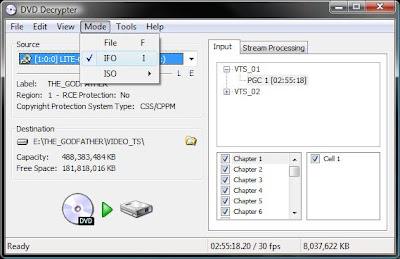3. In the information files list to your right, select the item that is the longest in case of a movie. In case of TV show, select the episode of interest.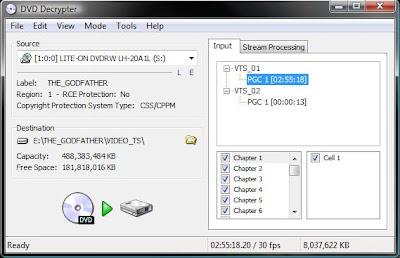4. Select the folder location of your decrypted DVD5. Finally, click on the decrypt button (with the green arrow)
Depending on the length of your DVD/episode and your platform, it usually takes less than 30 minutes to rip a 150 minutes DVD. When the decryption process in complete open up AutoGK and do the following
1. For your input file, choose the first VOB file in the extracted directory
2. Set the name and location of the output AVI
3. Select the first audio track
4. Select a subtitle (if any)
5. Select the output size. Usually, a 120 minutes DVD converts well to 700 MB. For 30 minute TV episodes, I would choose 175 MB while for a 1 hour episode, i would choose 350 MB. Alternatively, you can set the quality percentage. I find this the fastest way as AutoGK will perform a single pass. I usually keep this setting at around 65%
6. Click on advanced settings and choose Xvid and set the audio to VBR MP3 at 128 kbps . Note that you may need to download XviD (or DivX)
8. Click start
Voila!

Cite as:
Saad, T. "How to Rip a DVD into AVI". Weblog entry from Please Make A Note. https://pleasemakeanote.blogspot.com/2008/09/how-to-rip-dvd-into-avi.html

## Monday, September 1, 2008

### How to Post Math Equations in Blogger using ASCIIMathMIL

I know that most of us working directly with mathematics are looking for a fundamental solution to posting equations on blogger. So far, I would have my equations written in MathType, print the screen, paste, crop, and save in paint. It is a pain... a dual monitor setup would be helpful, nonethless, this is too much to go through.

I've seen several models over the net that provide different solutions, but none of them was what I was looking for, especially that I am not a LaTeX guru.

So far, the neatest model to post equations on blogger is described on Fundamental Thinking. The method uses Peter Jepsen's ASCIIMathML script.

Here's how to set it up on your blog:

1. Download ASCIIMathML.js and host it somewhere online such as your google pages website. This will allow you to modify the script to fit your needs (like changing the forecolor of the equations). Alternatively, you can just point to the location of the script (http://www1.chapman.edu/~jipsen/mathml/ASCIIMathML.js)
2. In Blogger, add a new HTML/Java Script widget
3. Add the following to the new widget
<script src="http://www1.chapman.edu/~jipsen/mathml/ASCIIMathML.js" type="text/javascript"></script>
(In case you decided to host the script on your own website, you will need to replace src with the correct location).
Voila!

Now you're all set. All you need to to do is either learn the ASCIIMathML syntax, type in LaTeX, or copy from Mathtype as LaTeX.

To type in LaTeX, you have to wrap your equations with a dollar sign.

Alternatively, you can use some online LaTeX editors such as Thornahawk's Numerical Analysis notes or CodeCogs', and then copy and paste the code into your post.

Finally, if you use MathType, you can set it up so that it copies the equations as LaTeX. Here's how you do it
1. In Mathtype, go to
Preferences/Translators
2. Select "Translation to other language"
3. Select "TeX -- LaTeX 2.09 and later"
4. Deselect "include MathType data in translation"
Make sure you replace the $and$ generated by MathType by the dollar sign.

This, I think, is by far the easiest way of getting your equations into blogger. Sometimes, the translation generated by MathType is not well interpreted by the ASCIIMathML script. For example, type the following in MathType

$\lim_{x\rightarrow 0}$

This will not be translated correctly with the ASCIIMathML.

After all this, I think we need a better solution.

UPDATE: As Bob Mathews from design science (the makers of MathType) has kindly noted, if you choose the Format/Inline Equation in MathType, the LaTeX code will exclude the $and$ symbols.

Cite as:
Saad, T. "How to Post Math Equations in Blogger using ASCIIMathMIL". Weblog entry from Please Make A Note. https://pleasemakeanote.blogspot.com/2008/09/how-to-post-math-equations-in-blogger.html

### How to Change the Manufacturer/OEM/Computer Logo in System Properties

In Windows Vista, there's usually a logo in the system properties (right click "My Computer"/Properties) that refers to the manufacturer of your machine. The original post can be found here. This can be easily changed by modifying the registry. Here's how
1. Create your own logo and save it as a bitmap (*.bmp) - Make sure the size is 120x120 pixels
2. Open the registry editor (click Start/regedit)
3. Go to:
HKEY_LOCAL_MACHINE \ SOFTWARE \ Microsoft \ Windows \ CurrentVersion \ OEMInformation
4. Modify the "Logo" entry &/ the "Manufacturer" entry
Voila!

Note that the "&/" symbol stand for "and, or".

Cite as:
Saad, T. "How to Change the Manufacturer/OEM/Computer Logo in System Properties". Weblog entry from Please Make A Note. https://pleasemakeanote.blogspot.com/2008/09/how-to-change-manufactureroemcomputer.html